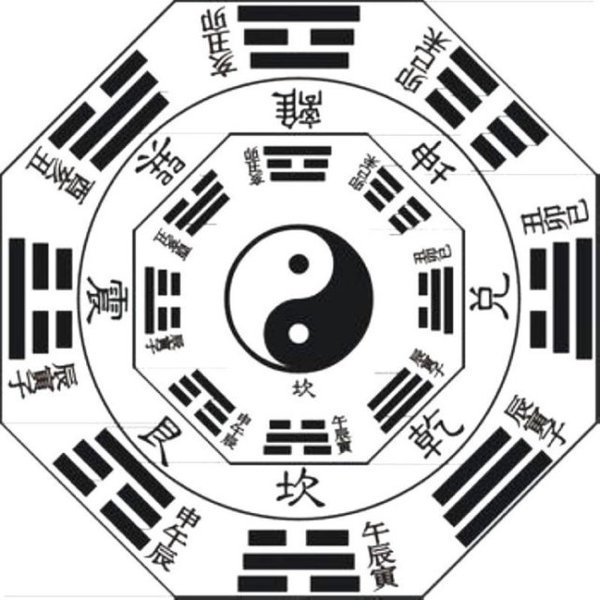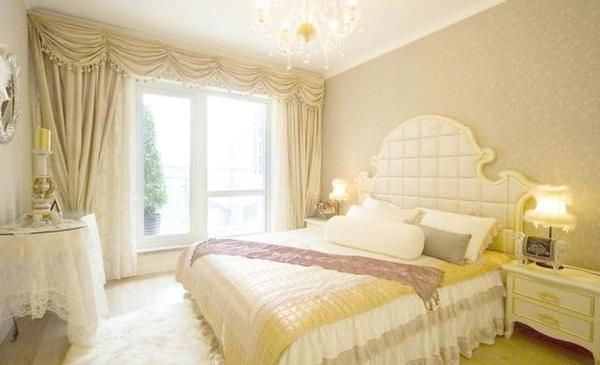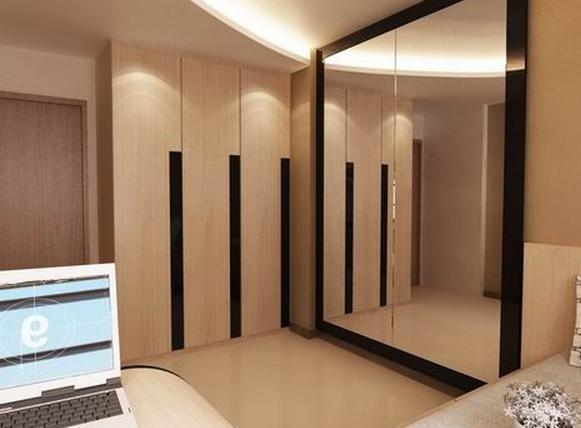|

# 家居风水原来这么重要!?风水学家居篇，卧室的风水学是很重要的一部分，作为每天呆的时间最长的地方，卧室的风水是很重要的，好的风水可以给人们带来好的影响。那么怎么创造一个好的卧室风水呢？昆明二十四城装饰拥有长期的装修经验，下面就是二十四城品推君给大家总结的主卧的风水禁忌：

主卧房不宜太亮：俗话说：“光厅暗房”意指不同于客厅风水之重明亮，卧房更看重的是浪漫协调，so千万不可光亮过重。如果太亮的话在外易犯小人，夫妻缠绵的情调也比较难以酝酿。家人健康容易受损，家庭成yuan的和谐也不容易保持，故仍是主卧居室风水大禁忌之一。

主卧房不宜太大或太小：主卧房如大过客厅，则容易在行事上易犯喧宾夺主的忌讳，导致是非口舌之祸，还会有部属背叛、诸事不顺等诸多不顺。如此说来如果主卧房太小，则男主人易有犯大男人主义的倾向，缺少应该具有的尊重与体贴。而女主人方面，则会容易变得有器量狭小的倾向，个人成就也会受到限制。床头不宜遭樑压：床头如遭樑压，则会使男主人或女主人之身体健康遭受损害，特别头部及神经系统容易出现问题，睡眠情况极度不佳，甚至还会影响到主人的工作运势，从而使得事业难以扩展。日常生活的压力不容易排除掉，忧郁加苦闷，难免会有志难伸之感。

床头不宜空荡：床头一定得有靠，亦即为床头背墙必为坚实的墙壁。床头定于玻璃之下，亦或者床头悬于空而无依靠，此乃风水之大忌讳。触犯者容易fa生婚姻之嫌隙，夫妻缘分淡薄，缺乏行之有效沟通，未婚者犯此孤相。卧室镜子的摆放—门边

卧室向来都是和我们最为息息相关的空间地点，卧室镜子的摆放一般可以放置在门旁，但是有一个问题需要我们注意的是，卧室镜子的摆放切记不能正对着卧室门，从风水学上说，镜子如果正对着门口则不利于家宅的和谐、安定，镜子正对着卧室门的话，我们很容易被自己的映像吓到，有次看来无论是风水学说还是日常需要注意的避讳，我们都不应该让镜子正对卧室摆放。卧室镜子的摆放—窗边

卧室镜子的摆放除了可以放置在门边，窗户旁边也应该是镜子放置的最佳场所，如果我们想将镜子放置在窗户附近的话，应该注意哪些忌讳呢？首先从实用性和观赏性方面考虑，其实卧室的镜子最好不要和窗户处于正对着的位置，镜子正对着窗户摆放，光线穿过过窗户反到镜子上会使我们使用起来极度不方便，且也不美观，我最好是将卧室镜子背靠着窗户放置，这样观赏性实用性是比较好的。

卧室镜子的摆放—卧室财位

在我们传统的风水学上认为，每一间卧室都有一个固定的点，这个点就成为空间中聚集财气与福气的地方，这些位置我们最好注意避开任何带有反射、折射效果的物品，像镜子之类的东西就不要放置在我们的卧室财位位置。那大家可能就要想了，什么样的位置才算是卧室的财位位置呢？以我们进入主卧室的门为一个基点，它的左右方向对角线的位置就是我是财位位置，卧室镜子摆放切千万不要选择这个地方哦！卧室镜子的摆放—镜子宜明

那么在对卧室镜子做摆放研究的时候，可能会考虑到各种方位问题，对于光线和视觉效果大家是否做过特别的关注呢？就按实际情况来说，卧室的镜子应该最好是放置在比较通达、明亮的位置最好，而从风水学上讲，镜子应该明亮而不应该黯淡，这也有着一定得科学依据。环境过暗会使镜子使用效果不佳，况且模糊不清晰的镜像很容易让人不经意间吓坏自己，因此卧室的镜子最好应该是放置在光线较好的角落。

卧室镜子的摆放—圆形镜子

现在市面上的镜子样式和形式繁多，不管是什么材质、什么样式的卧室镜子，我们都能够看到。在各种各样的镜子产品中，我们要如何选择比较适合自己卧室的镜子呢，且在卧室镜子的摆放上，镜子的形状都有什么样的要求呢？按老一辈的说法，镜子最好是圆形的，象征着家庭幸福、美满，其次镜子最好带有镶边，这样就不会使得财气外泄，圆润、光亮的镜子也预兆着过我们带来光亮与希望。

卧室镜子的摆放—厕所

若是卧室中带有厕所，镜子的摆放位置一定要注意，千万不要将镜子摆放在对着厕所门的地方，如果镜子对着厕所门，容易让两夫妻在处理事情的时后钻牛角尖。`声明：本文由入驻焦点开放平台的作者撰写，除焦点官方账号外，观点仅代表作者本人，不代表焦点立场错误信息举报电话： 400-099-0099，邮箱：jubao@vip.sohu.com，或点此进行意见反馈，或点此进行举报投诉。`A B C D E F G H J K L M N P Q R S T W X Y Z
A - B - C - D - E
• A
• 鞍山
• 安庆
• 安阳
• 安顺
• 安康
• 澳门
• B
• 北京
• 保定
• 包头
• 巴彦淖尔
• 本溪
• 蚌埠
• 亳州
• 滨州
• 北海
• 百色
• 巴中
• 毕节
• 保山
• 宝鸡
• 白银
• 巴州
• C
• 承德
• 沧州
• 长治
• 赤峰
• 朝阳
• 长春
• 常州
• 滁州
• 池州
• 长沙
• 常德
• 郴州
• 潮州
• 崇左
• 重庆
• 成都
• 楚雄
• 昌都
• 慈溪
• 常熟
• D
• 大同
• 大连
• 丹东
• 大庆
• 东营
• 德州
• 东莞
• 德阳
• 达州
• 大理
• 德宏
• 定西
• 儋州
• 东平
• E
• 鄂尔多斯
• 鄂州
• 恩施
F - G - H - I - J
• F
• 抚顺
• 阜新
• 阜阳
• 福州
• 抚州
• 佛山
• 防城港
• G
• 赣州
• 广州
• 桂林
• 贵港
• 广元
• 广安
• 贵阳
• 固原
• H
• 邯郸
• 衡水
• 呼和浩特
• 呼伦贝尔
• 葫芦岛
• 哈尔滨
• 黑河
• 淮安
• 杭州
• 湖州
• 合肥
• 淮南
• 淮北
• 黄山
• 菏泽
• 鹤壁
• 黄石
• 黄冈
• 衡阳
• 怀化
• 惠州
• 河源
• 贺州
• 河池
• 海口
• 红河
• 汉中
• 海东
• 怀来
• I
• J
• 晋中
• 锦州
• 吉林
• 鸡西
• 佳木斯
• 嘉兴
• 金华
• 景德镇
• 九江
• 吉安
• 济南
• 济宁
• 焦作
• 荆门
• 荆州
• 江门
• 揭阳
• 金昌
• 酒泉
• 嘉峪关
K - L - M - N - P
• K
• 开封
• 昆明
• 昆山
• L
• 廊坊
• 临汾
• 辽阳
• 连云港
• 丽水
• 六安
• 龙岩
• 莱芜
• 临沂
• 聊城
• 洛阳
• 漯河
• 娄底
• 柳州
• 来宾
• 泸州
• 乐山
• 六盘水
• 丽江
• 临沧
• 拉萨
• 林芝
• 兰州
• 陇南
• M
• 牡丹江
• 马鞍山
• 茂名
• 梅州
• 绵阳
• 眉山
• N
• 南京
• 南通
• 宁波
• 南平
• 宁德
• 南昌
• 南阳
• 南宁
• 内江
• 南充
• P
• 盘锦
• 莆田
• 平顶山
• 濮阳
• 攀枝花
• 普洱
• 平凉
Q - R - S - T - W
• Q
• 秦皇岛
• 齐齐哈尔
• 衢州
• 泉州
• 青岛
• 清远
• 钦州
• 黔南
• 曲靖
• 庆阳
• R
• 日照
• 日喀则
• S
• 石家庄
• 沈阳
• 双鸭山
• 绥化
• 上海
• 苏州
• 宿迁
• 绍兴
• 宿州
• 三明
• 上饶
• 三门峡
• 商丘
• 十堰
• 随州
• 邵阳
• 韶关
• 深圳
• 汕头
• 汕尾
• 三亚
• 三沙
• 遂宁
• 山南
• 商洛
• 石嘴山
• T
• 天津
• 唐山
• 太原
• 通辽
• 铁岭
• 泰州
• 台州
• 铜陵
• 泰安
• 铜仁
• 铜川
• 天水
• 天门
• W
• 乌海
• 乌兰察布
• 无锡
• 温州
• 芜湖
• 潍坊
• 威海
• 武汉
• 梧州
• 渭南
• 武威
• 吴忠
• 乌鲁木齐
X - Y - Z
• X
• 邢台
• 徐州
• 宣城
• 厦门
• 新乡
• 许昌
• 信阳
• 襄阳
• 孝感
• 咸宁
• 湘潭
• 湘西
• 西双版纳
• 西安
• 咸阳
• 西宁
• 仙桃
• 西昌
• Y
• 运城
• 营口
• 盐城
• 扬州
• 鹰潭
• 宜春
• 烟台
• 宜昌
• 岳阳
• 益阳
• 永州
• 阳江
• 云浮
• 玉林
• 宜宾
• 雅安
• 玉溪
• 延安
• 榆林
• 银川
• Z
• 张家口
• 镇江
• 舟山
• 漳州
• 淄博
• 枣庄
• 郑州
• 周口
• 驻马店
• 株洲
• 张家界
• 珠海
• 湛江
• 肇庆
• 中山
• 自贡
• 资阳
• 遵义
• 昭通
• 张掖
• 中卫

1室1厅1厨1卫1阳台

1
2
3
4
5

0
1
2

1

1

0
1
2
3报名成功，资料已提交审核A B C D E F G H J K L M N P Q R S T W X Y Z
A - B - C - D - E
• A
• 鞍山
• 安庆
• 安阳
• 安顺
• 安康
• 澳门
• B
• 北京
• 保定
• 包头
• 巴彦淖尔
• 本溪
• 蚌埠
• 亳州
• 滨州
• 北海
• 百色
• 巴中
• 毕节
• 保山
• 宝鸡
• 白银
• 巴州
• C
• 承德
• 沧州
• 长治
• 赤峰
• 朝阳
• 长春
• 常州
• 滁州
• 池州
• 长沙
• 常德
• 郴州
• 潮州
• 崇左
• 重庆
• 成都
• 楚雄
• 昌都
• 慈溪
• 常熟
• D
• 大同
• 大连
• 丹东
• 大庆
• 东营
• 德州
• 东莞
• 德阳
• 达州
• 大理
• 德宏
• 定西
• 儋州
• 东平
• E
• 鄂尔多斯
• 鄂州
• 恩施
F - G - H - I - J
• F
• 抚顺
• 阜新
• 阜阳
• 福州
• 抚州
• 佛山
• 防城港
• G
• 赣州
• 广州
• 桂林
• 贵港
• 广元
• 广安
• 贵阳
• 固原
• H
• 邯郸
• 衡水
• 呼和浩特
• 呼伦贝尔
• 葫芦岛
• 哈尔滨
• 黑河
• 淮安
• 杭州
• 湖州
• 合肥
• 淮南
• 淮北
• 黄山
• 菏泽
• 鹤壁
• 黄石
• 黄冈
• 衡阳
• 怀化
• 惠州
• 河源
• 贺州
• 河池
• 海口
• 红河
• 汉中
• 海东
• 怀来
• I
• J
• 晋中
• 锦州
• 吉林
• 鸡西
• 佳木斯
• 嘉兴
• 金华
• 景德镇
• 九江
• 吉安
• 济南
• 济宁
• 焦作
• 荆门
• 荆州
• 江门
• 揭阳
• 金昌
• 酒泉
• 嘉峪关
K - L - M - N - P
• K
• 开封
• 昆明
• 昆山
• L
• 廊坊
• 临汾
• 辽阳
• 连云港
• 丽水
• 六安
• 龙岩
• 莱芜
• 临沂
• 聊城
• 洛阳
• 漯河
• 娄底
• 柳州
• 来宾
• 泸州
• 乐山
• 六盘水
• 丽江
• 临沧
• 拉萨
• 林芝
• 兰州
• 陇南
• M
• 牡丹江
• 马鞍山
• 茂名
• 梅州
• 绵阳
• 眉山
• N
• 南京
• 南通
• 宁波
• 南平
• 宁德
• 南昌
• 南阳
• 南宁
• 内江
• 南充
• P
• 盘锦
• 莆田
• 平顶山
• 濮阳
• 攀枝花
• 普洱
• 平凉
Q - R - S - T - W
• Q
• 秦皇岛
• 齐齐哈尔
• 衢州
• 泉州
• 青岛
• 清远
• 钦州
• 黔南
• 曲靖
• 庆阳
• R
• 日照
• 日喀则
• S
• 石家庄
• 沈阳
• 双鸭山
• 绥化
• 上海
• 苏州
• 宿迁
• 绍兴
• 宿州
• 三明
• 上饶
• 三门峡
• 商丘
• 十堰
• 随州
• 邵阳
• 韶关
• 深圳
• 汕头
• 汕尾
• 三亚
• 三沙
• 遂宁
• 山南
• 商洛
• 石嘴山
• T
• 天津
• 唐山
• 太原
• 通辽
• 铁岭
• 泰州
• 台州
• 铜陵
• 泰安
• 铜仁
• 铜川
• 天水
• 天门
• W
• 乌海
• 乌兰察布
• 无锡
• 温州
• 芜湖
• 潍坊
• 威海
• 武汉
• 梧州
• 渭南
• 武威
• 吴忠
• 乌鲁木齐
X - Y - Z
• X
• 邢台
• 徐州
• 宣城
• 厦门
• 新乡
• 许昌
• 信阳
• 襄阳
• 孝感
• 咸宁
• 湘潭
• 湘西
• 西双版纳
• 西安
• 咸阳
• 西宁
• 仙桃
• 西昌
• Y
• 运城
• 营口
• 盐城
• 扬州
• 鹰潭
• 宜春
• 烟台
• 宜昌
• 岳阳
• 益阳
• 永州
• 阳江
• 云浮
• 玉林
• 宜宾
• 雅安
• 玉溪
• 延安
• 榆林
• 银川
• Z
• 张家口
• 镇江
• 舟山
• 漳州
• 淄博
• 枣庄
• 郑州
• 周口
• 驻马店
• 株洲
• 张家界
• 珠海
• 湛江
• 肇庆
• 中山
• 自贡
• 资阳
• 遵义
• 昭通
• 张掖
• 中卫• 手机• 分享
• 设计
免费设计
• 计算器
装修计算器
• 入驻
合作入驻
• 联系
联系我们
• 置顶
返回顶部